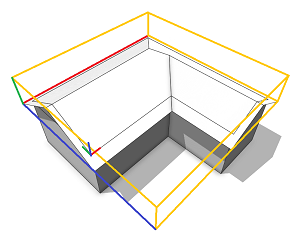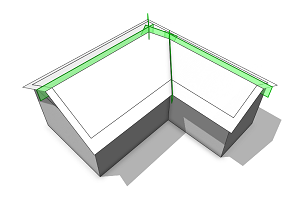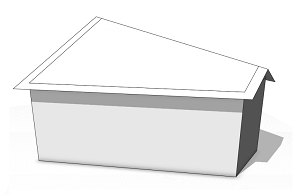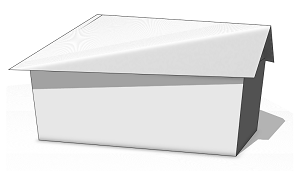# roofGable

### Syntax

• roofGable(angle)
• roofGable(angle, overhangX)
• roofGable(angle, overhangX, overhangY)
• roofGable(angle, overhangX, overhangY, even)
• roofGable(angle, overhangX, overhangY, even, index)
• roofGable(valueType, value)
• roofGable(valueType, value, overhangX)
• roofGable(valueType, value, overhangX, overhangY)
• roofGable(valueType, value, overhangX, overhangY, even)

### Parameters

1. anglefloat
Angle of the roof-planes (generation byAngle).
2. overhangXfloat
Overhang distance for overhangs perpendicular to ridges, measured perpendicular to the shape edges (on the roof).
3. overhangYfloat
Overhang distance for overhangs in the direction of the ridges, measured perpendicular to the shape edges (on the roof).
4. evenbool
Whether to make the roof gable even or not. If true, non-planar faces can originate.
5. indexfloat
Edge index to control the orientation of the ridge.
6. valueTypeselector
{ byAngle | byHeight }—Type of roof generation.
7. valuefloat
Angle or height of the roof-planes as specified by valueType.

### Description

The roofGable operation builds a gable roof perpendicular to each face of the current shape’s geometry. The orientation of the ridges is automatically computed (except in the indexed case). At all non-ridge (eave) edges, a plane is generated with a given angle or height wrt. the polygon plane. The planes are cut with each other to form the roof faces:

• If overhangX is set, the roof faces overlap along the eave edges by this distance. Overhang distances are measured perpendicular to the edges (on the roof planes).
• If overhangY is set, the roof faces overlap in the direction of the ridges by this distance. Overhang distances are measured perpendicular to the shape edges (on the roof planes).
• If even is set to true, the gable edges are forced to be horizontal. In this case, non-planar roof faces can originate.
• If index is set, the ridge is forced to be oriented in the direction of the edge index.
##### Caution:

This only works on convex shapes with a single face. The even parameter is neglected.

##### Note:

The connectivity of the roof mesh is optimized for trim plane generation to cut bricks inserted into the roof planes (see examples below).

### Scope

The scope orientation is set in the following way:

• x-axis direction is kept as much as possible (old x-axis is projected to the plane of the first face).
• y-axis along the face normal of the first face.
• z-axis normal to the two above.

The scope’s sizes are adjusted to tightly fit the extruded geometry.

### Examples

#### Simple Gable Roof

A basic gable roof is generated on top of an extruded L-lot.

 A gable roof with roof slope 30 degrees is built on top of an extruded L-lot. The overhangX distance is set to 2 and theoverhangY distance is set to 1. Note the different overlap distances at the eaves (X) and in the direction of the ridges (Y). Also note the setting of the pivot and scope. ``````Lot --> extrude(10) Mass Mass --> comp(f) { top : Top | all : X } Top --> roofGable(30, 2, 1) Roof``````After a component split, each roof face contains trim planes to cut bricks on insertion. Here, per default there are no horizontal trim planes at the ridges. To enable them, set(trim.horizontal, true) is used in front of the component split (details). ``````Roof --> set(trim.horizontal, true) comp(f) { all : X }``````##### Note:

There is exactly one roof face per Top shape edge. Unfortunately, in the images it seems like the overlap is in a separate face. However, the simple reason for this is that the edges of the faces in behind bleed through.

#### Even Gable Roof

This example demonstrates the difference between a standard and an even gable roof built on a trapezoid-lot.

 A gable roof with roof slope 30 degrees is built on top of an extruded trapezoid-lot. The overhangs (X and Y) are both set to 1. Note that the ridge is uneven. ``````Lot --> extrude(10) Mass Mass --> comp(f) { top : Top | all : X } Top --> roofGable(30, 1, 1, false) Roof``````When using the above rule for the Top shape, the ridge vertices are set to the average height, making the gable roof even. The roofs are non-planar now. ``````Top --> roofGable(30, 1, 1, true) Roof``````##### Note:

For many shapes, ridges get implicitly even and hence the even parameter doesn’t change anything.